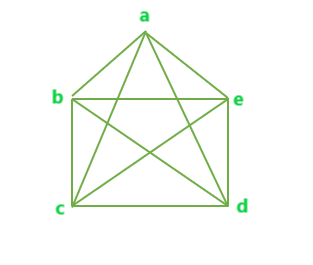# Program to find total number of edges in a Complete Graph

Given N number of vertices of a Graph. The task is to find the total number of edges possible in a complete graph of N vertices.

Complete Graph: A Complete Graph is a graph in which every pair of vertices is connected by an edge.

Examples:

```Input : N = 3
Output : Edges = 3

Input : N = 5
Output : Edges = 10
```

## Recommended: Please try your approach on {IDE} first, before moving on to the solution.

The total number of possible edges in a complete graph of N vertices can be given as,

Total number of edges in a complete graph of N vertices = ( n * ( n – 1 ) ) / 2

Example 1: Below is a complete graph with N = 5 vertices.The total number of edges in the above complete graph = 10 = (5)*(5-1)/2.

Below is the implementation of the above idea:

## C++

 `// C++ implementation to find the ` `// number of edges in a complete graph ` ` `  `#include ` `using` `namespace` `std; ` ` `  `// Function to find the total number of ` `// edges in a complete graph with N vertices ` `int` `totEdge(``int` `n) ` `{ ` `    ``int` `result = 0; ` ` `  `    ``result = (n * (n - 1)) / 2; ` ` `  `    ``return` `result; ` `} ` ` `  `// Driver Code ` `int` `main() ` `{ ` `    ``int` `n = 6; ` ` `  `    ``cout << totEdge(n); ` ` `  `    ``return` `0; ` `} `

## Java

 `// Java implementation to find the ` `// number of edges in a complete graph ` ` `  `class` `GFG { ` `     `  `// Function to find the total number of ` `// edges in a complete graph with N vertices ` `static` `int` `totEdge(``int` `n) ` `{ ` `    ``int` `result = ``0``; ` ` `  `    ``result = (n * (n - ``1``)) / ``2``; ` ` `  `    ``return` `result; ` `} ` ` `  `    ``// Driver Code ` `    ``public` `static` `void` `main(String []args) ` `    ``{ ` `        ``int` `n = ``6``; ` `        ``System.out.println(totEdge(n)); ` `    ``} ` ` `  `} `

## Python 3

 `# Python 3 implementation to   ` `# find the number of edges  ` `# in a complete graph  ` ` `  `# Function to find the total  ` `# number of edges in a complete ` `# graph with N vertices ` `def` `totEdge(n) : ` ` `  `    ``result ``=` `(n ``*` `(n ``-` `1``)) ``/``/` `2` ` `  `    ``return` `result ` `             `  `# Driver Code ` `if` `__name__ ``=``=` `"__main__"` `: ` ` `  `    ``n ``=` `6` ` `  `    ``print``(totEdge(n)) ` ` `  `# This code is contributed ` `# by ANKITRAI1 `

## C#

 `// C# implementation to find  ` `// the number of edges in a ` `// complete graph ` `using` `System; ` ` `  `class` `GFG  ` `{ ` `     `  `// Function to find the total  ` `// number of edges in a complete  ` `// graph with N vertices ` `static` `int` `totEdge(``int` `n) ` `{ ` `    ``int` `result = 0; ` ` `  `    ``result = (n * (n - 1)) / 2; ` ` `  `    ``return` `result; ` `} ` ` `  `// Driver Code ` `public` `static` `void` `Main() ` `{ ` `    ``int` `n = 6; ` `    ``Console.Write(totEdge(n)); ` `} ` `} ` ` `  `// This code is contributed  ` `// by ChitraNayal `

## PHP

 ` `

Output:

```15
```

Don’t stop now and take your learning to the next level. Learn all the important concepts of Data Structures and Algorithms with the help of the most trusted course: DSA Self Paced. Become industry ready at a student-friendly price.

My Personal Notes arrow_drop_upCheck out this Author's contributed articles.

If you like GeeksforGeeks and would like to contribute, you can also write an article using contribute.geeksforgeeks.org or mail your article to contribute@geeksforgeeks.org. See your article appearing on the GeeksforGeeks main page and help other Geeks.

Please Improve this article if you find anything incorrect by clicking on the "Improve Article" button below.

Article Tags :
Practice Tags :

2

Please write to us at contribute@geeksforgeeks.org to report any issue with the above content.